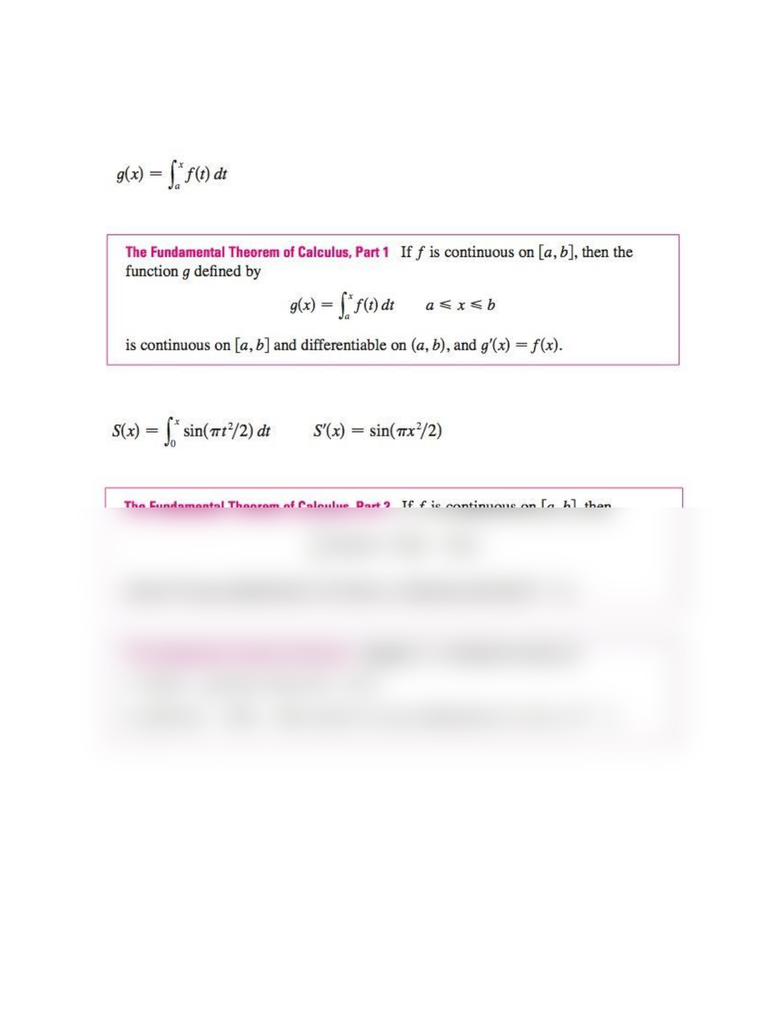Textbook Notes (280,000)
US (110,000)
UCSB (700)
MATH 3B (10)
helms (10)
Chapter 5.3

# MATH 3B Chapter Notes - Chapter 5.3: Antiderivative

This preview shows half of the first page. to view the full 1 pages of the document.Math 3B: Ch. 5.3 The Fundamental Theorem of Calculus
functions defined by an equation in the form
Fresnel function: